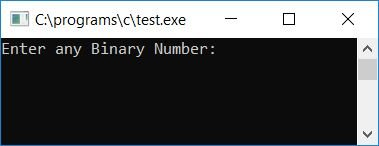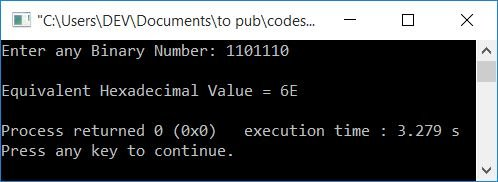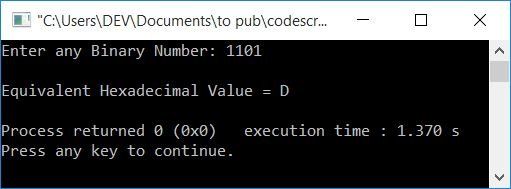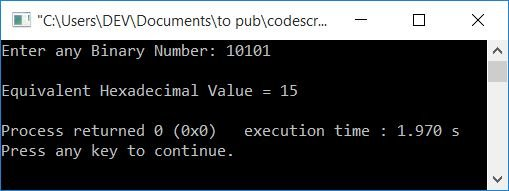# C Program to Convert Binary to Hexadecimal

In this article, we will learn how to create a program in C that can convert any given binary number entered by the user (at run-time) into its equivalent hexadecimal value. At last, we have also created a function-driven program that also converts binary numbers to hexadecimal numbers.

But before going through the program, if you are not aware of how the binary to hexadecimal conversion takes place, refer to the step-by-step process of binary to hexadecimal conversion. Now let's move on to the program.

## Binary to Hexadecimal in C

The question is, "Write a program in C that converts a binary number to hexadecimal." The answer to this question is:

```#include<stdio.h>
#include<conio.h>
int main()
{
int binnum, hex=0, mul=1, count=1, rem, i=0;
char hexnum;
printf("Enter any Binary Number: ");
scanf("%d", &binnum);
while(binnum!=0)
{
rem = binnum%10;
hex = hex + (rem*mul);
if(count%4==0)
{
if(hex<10)
hexnum[i] = hex+48;
else
hexnum[i] = hex+55;
mul = 1;
hex = 0;
count = 1;
i++;
}
else
{
mul = mul*2;
count++;
}
binnum = binnum/10;
}
if(count!=1)
hexnum[i] = hex+48;
if(count==1)
i--;
for(i=i; i>=0; i--)
printf("%c", hexnum[i]);
getch();
return 0;
}```

Because the above program was written in the Code::Blocks IDE, you will see the following output on your output screen after a successful build and run. This is the first snapshot of the sample run:Now supply any binary number, say 1101110, and press the ENTER key to see its equivalent hexadecimal value, as shown in the second snapshot of the sample run given below:Here is the final snapshot of the above program's another sample run:#### Program Explained

• Receive any binary number from the user at run-time, say 1101.
• Create a while loop that runs until the value of the given binary number becomes 0.
• That is, at the first run of the while loop, the condition binnum!=0 or 1101!=0 evaluates to true, therefore program flow goes inside the loop and binnum%10 or 1101%10 or 1 gets initialized to rem, then hex + (rem*mul) (we initialized hex and mul with 0 and 1 at the start of the program) or 0 + (1*1) or 1 gets initialized to hex.
• Now we have created an if block in such a way that the value of hex gets initialized to hexnum[], a character array as hexadecimal digits, one by one, in corresponding to each 4-pair binary digit.
• Therefore, at the first run of the while loop, the condition of the if statement, which is count%4==0 (we have initialized count with 1 at the start of the program) or 1%4==0, evaluates to false, and therefore program flow goes inside the else block of the if statement, where mul*2 or 1*2 or 2 gets initialized to mul, and the value of the count variable gets incremented and becomes 2.
• At last, binnum/10 or 1101/10 or 110 gets initialized to binnum, and then the program flow goes back to the condition of the while loop.
• There, again, binnum!=0 or 110!=0 evaluates to true, and the program flow again goes inside the while loop.
• And binnum%10 or 110%10 or 0 gets initialized to rem, then hex + (rem*mul) or 1 + (0*2) or 1 gets initialized to hex.
• Now the condition of the if block, which is count%4==0 or 2%4==0 evaluates to false, and the program flow goes inside the else block, where mul*2 or 2*2 or 4 gets initialized to mul and the value of count gets incremented and becomes 3.
• At last, binnum/10 or 110/10 or 11 gets initialized to binnum, and the program flow goes back to the condition of the while loop.
• There, again, binnum!=0 or 11!=0 evaluates to true, and the program flow again goes inside the while loop.
• And binnum%10 or 11%10 or 1 gets initialized to rem, then hex + (rem*mul) or 1 + (1*4) or 5 gets initialized to hex.
• Now the condition of the if block, which is count%4==0 or 3%4==0, evaluates to false, and the program flow goes inside the else block, and mul*2 or 4*2 or 8 gets initialized to mul, and the value of count gets incremented and becomes 4.
• At last, binnum/10, 11/10, or 1 gets initialized to binnum, and then the program flow goes back to the condition of the while loop.
• There, again, binnum!=0 or 1!=0 evaluates to true, and the program flow again goes inside the while loop.
• And binnum%10 or 1%10 or 1 gets initialized to rem, then hex + (rem*mul) or 5 + (1*8) or 13 gets initialized to hex.
• Now that the condition of the if block, count%4==0 or 4%4==0, evaluates to true, the program flow returns to the if block and because the value of hex (13) is greater than 10, hex+55 or 13+55 or 68 (68 is the ASCII code for the character "C") gets set to hexnum[i] (we set the variable i to 0 at the start of the program) or hexnum, and then 1, 0, and 1 are set to mul, hex, and count, respectively. And the value of i gets incremented and becomes 1.
• At last, binnum/10, 1/10, or 0, gets initialized to binnum, and the program flow goes back to the condition of the while loop.
• There, binnum!=0 or 0!=0 evaluates to false, and the program flow does not go inside the loop this time, that is, the program flow exits the loop, and then the condition count!=1 or 1!=1 evaluates to false, therefore the program flow does not go inside the if block.
• Because the condition of the second if block, count==1 or 1==1 evaluates to true, the value of i gets decremented by one and becomes 0.
• Now we have created the for loop that runs from the current value of i to 0.
• And print the value of each and every hexadecimal digit one by one, from the last index to the first one.
• For the numbers and characters, we used ASCII code, which is 0, 1, 2, 3,..., 7, 8, 9, A, B, C, D, E, F.
• That is, the ASCII codes for 0, 1, 2,..., 8, 9, are 48, 49, 50,..., 56, 57. In addition, the ASCII codes for A, B, C,..., E, F are 65, 66, 67,..., 69, 70.
• As a result, if the value of hex is 0, then 0+48 (the ASCII code for 0) is initialized to hexnum[; similarly, if the value of hex is 13, then 13+55 or 68 (the ASCII code for C) is initialized to hexnum[].

### Binary to Hexadecimal in C using a User-Defined Function

Here is another program using a user-defined function named BinToHex() that also does the same job, which is to convert any binary number to a hexadecimal number.

Here, we have declared the variable i and the character array hexnum[] as global to make them known in both the functions, that is, main() and  BinToHex().

```#include<stdio.h>
#include<conio.h>
void BinToHex(int bin);
int i=0;
char hexnum;
int main()
{
int binnum;
printf("Enter any Binary Number: ");
scanf("%d", &binnum);
BinToHex(binnum);
for(i=i; i>=0; i--)
printf("%c", hexnum[i]);
getch();
return 0;
}
void BinToHex(int bin)
{
int hex=0, mul=1, count=1, rem;
while(bin!=0)
{
rem = bin%10;
hex = hex + (rem*mul);
if(count%4==0)
{
if(hex<10)
hexnum[i] = hex+48;
else
hexnum[i] = hex+55;
mul = 1;
hex = 0;
count = 1;
i++;
}
else
{
mul = mul*2;
count++;
}
bin = bin/10;
}
if(count!=1)
hexnum[i] = hex+48;
if(count==1)
i--;
}```

Here is the final snapshot of the above program's sample run:#### The same program in different languages

C Quiz

« Previous Program Next Program »